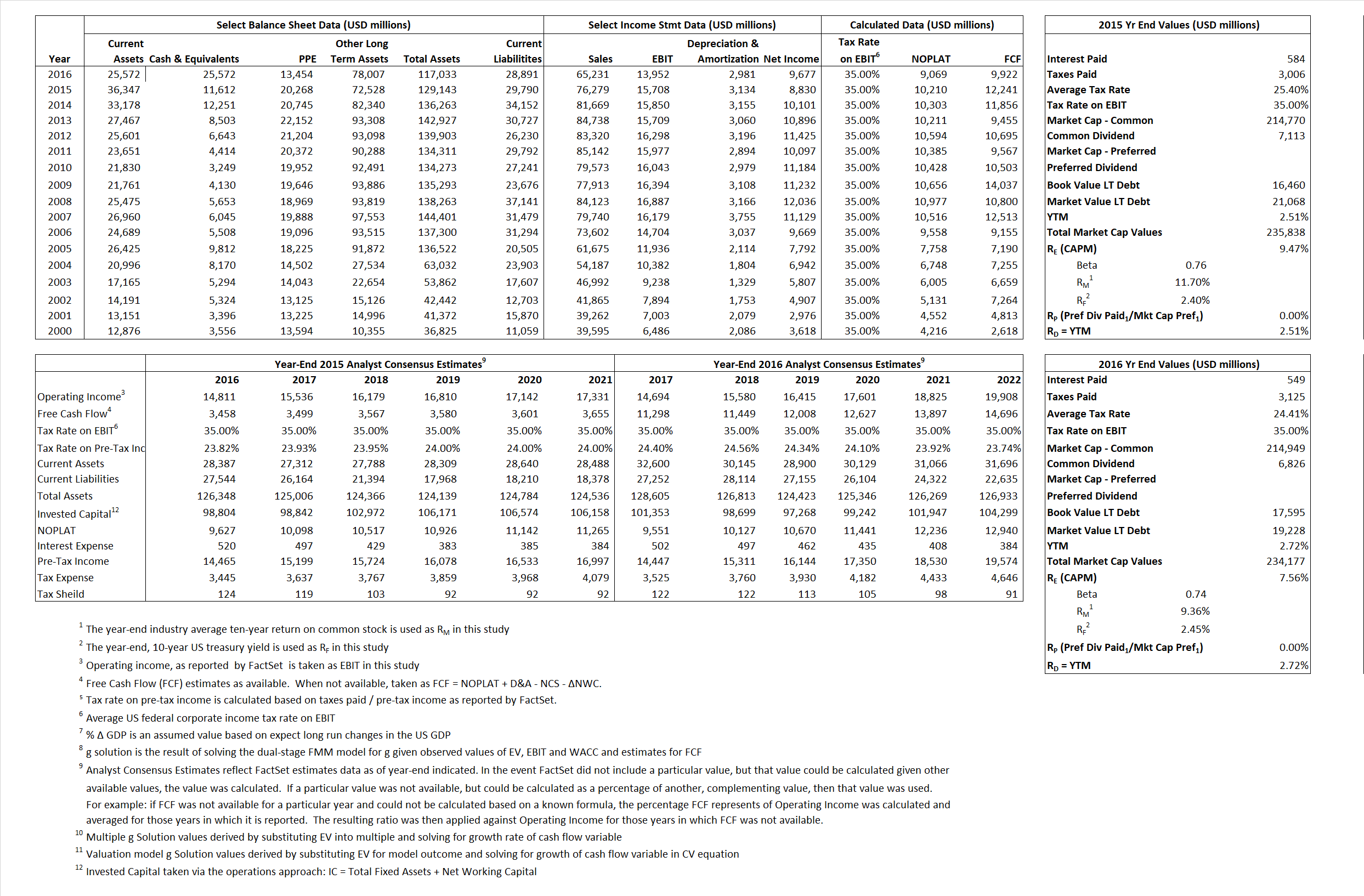# Procter & Gamble Co

## Analyst Listing

The following analysts provide coverage for the subject firm as of May 2016:

 Broker Analyst Analyst Email Bernstein Research Ali Dibadj ali.dibadj@bernstein.com CRT Capital Group April Scree ascee@sterneageecrt.com Deutsche Bank Research Bill Schmitz Jr. william.schmitz@db.com Atlantic Equities Charles Eden c.eden@atlantic-equities.com Consumer Edge Research Javier Escalante jescalante@consumeredgeresearch.com Hilliard Lyons Jeffrey S. Thomison jthomison@hilliard.com William Blair Jon R. Andersen jandersen@williamblair.com Raymond James Joseph Altobello joe.altobello@raymondjames.com B Riley & Co Linda Bolton Weiser lweiser@brileyco.com Stifel Nicolaus Mark S. Astrachan msastrachan@stifel.com RBC Capital Markets Nik Modi nik.modi@rbccm.com SunTrust Robinson Humphrey William B. Chappell Jr. bill.chappell@suntrust.com

## Primary Input Data## Derived Input Data

### Equational Form

Net Operating Profit Less Adjusted Taxes NOPLAT  10,210  9,069$NOPLAT\, =\, EBIT\, x\, (1 \,-\, Avg \,\,Tax\,\, Rate\,\, on\,\, EBIT)$
Free Cash Flow FCF 12,241 9,922$FCF\,=NOPLAT\,+\,Non-Cash\,Expenses-\Delta NWC\,-\,NCS$
Tax Shield TS 148 134$TS\,=\,Interest\,\,Paid\,\,x\,\, Avg \,\,Tax\,\,Rate\,\, on\,\, Pre-Tax\,\, Income$
Invested Capital IC 99,353 88,142$IC\,=\,Fixed\,\,Operating\,\,Assets\,\,+\,\,Net\,\, Working\,\, Capital$
Return on Invested Capital ROIC 10.28% 10.29%$ROIC\,=\,\frac { NOPLAT }{ IC }$
Net Investment NetInv 376 (8,230)$NetInv\,=\,{ {IC}_{1}}-{{IC}_{0}}+Depreciation$
Investment Rate IR 3.68% -90.75%$IR\,=\,\frac {NetInv}{NOPLAT}$
Weighted Average Cost of Capital
WACCMarket 8.79% 7.11%$WACC\,=\,\frac { E }{ V } { R }_{ E }\,+\,\frac { P }{ V } { R }_{ P }\,+\,\frac { D }{ V } { R }_{ D }\left( 1- Avg\,\, Tax\,\,Rate\,\,on\,\,Pre-Tax\,\,Income \right)$
WACCBook  6.62%   6.68%
Enterprise value
EVMarket 224,226 208,605$EV\,=\,Market\,\,Cap\,\,Equity\,+\,\,Long\,\,Term\,\,Debt\,-\,Cash$
EVBook  205,658  206,972
Long-Run Growth
g = IR x ROIC
0.38%  -9.34% Long-run growth rates of the income variable are used in the Continuing Value portion of the valuation models.
g = %$\Delta$ GDP   2.50%   2.50%
Margin from Operations M   20.59%  21.39%$M\,\,=\,\,\frac{EBIT}{SALES}$
Depreciation/Amortization Rate D   16.63%  17.60%$D\,\,=\,\,\frac{D+A}{EBITDA}$

## Valuation Multiple Outcomes

The outcomes presented in this study are the result of original input data, derived data, and synthesized inputs.

### model g solution

12/31/2015 12/31/2016 12/31/2015 12/31/2016 12/31/2015 12/31/2016

EV/SALES$\frac {EV}{Sales} \,= \,\frac{ROIC\, -\, g}{ROIC\,(WACC\,-\,g)}\,(1\,-\,T)\,(M)$

2.94  3.20  7.61% 4.79%  8.12%  5.75%

EV/EBITDA$\frac {EV}{EBITDA} \,= \,\frac{ROIC\, -\, g}{ROIC\,(WACC\,-\,g)}\,(1\,-\,T)\,(1\,-\,D)$

11.90 12.32 7.61% 4.79% 8.12% 5.75%

EV/NOPLAT$\frac {EV}{NOPLAT} \,= \,\frac{ROIC\, -\, g}{ROIC\,(WACC\,-\,g)}$

21.96 23.00 7.61% 4.79% 8.12% 5.75%

EV/FCFOPS$\frac {EV}{FCF_{OPS}} \,= \,\frac{ROIC\, -\, g}{ROIC\,(WACC\,-\,g)}\,(1\,-\,T)$

18.32 21.02 7.61% 4.79% 8.12% 5.75%

EV/EBIT$\frac {EV}{EBIT} \,= \,\frac{ROIC\, -\, g}{ROIC\,(WACC\,-\,g)}\,(1\,-\,T)$

14.27 14.95 7.61% 4.79% 8.12% 5.75%

EV/IC$\frac {EV}{IC} \,= \,\frac{ROIC\, -\, g}{WACC\,-\,g}$

2.26 2.37 7.61% 4.79%  8.12% 5.75%